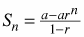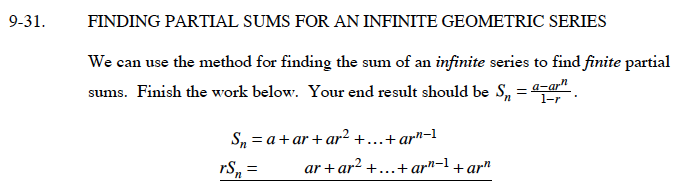### Home > CALC > Chapter 9 > Lesson 9.1.2 > Problem9-31

9-31.

FINDING PARTIAL SUMS FOR AN INFINITE GEOMETRIC SERIES
We can use the method for finding the sum of an infinite series to find finite partial sums. Finish the work below. Your end result should be. Homework Help ✎Factor the left side of the equation.